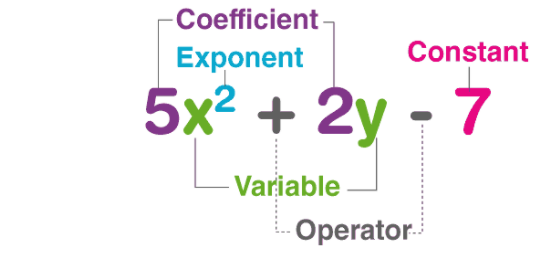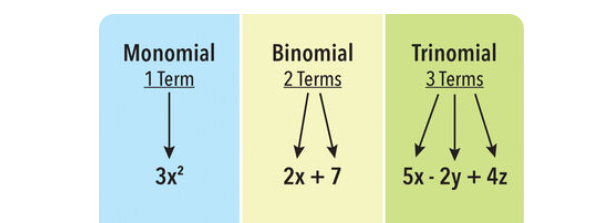•Sign In
• Hire UsUh Oh! It seems you’re using an Ad blocker!

We always struggled to serve you with the best online calculations, thus, there's a humble request to either disable the AD blocker or go with premium plans to use the AD-Free version for calculators.

Or# Multiplying Polynomials Calculator

Write down two polynomials and the calculator will find their product, with detailed calculations shown.

Enter First Polynomial:

Enter Second Polynomial:

Table of Content

 1 What is priceeight Class? 2 priceeight Class Chart: 3 How to Calculate priceeight Density (Step by Step): 4 Factors that Determine priceeight Classification: 5 What is the purpose of priceeight Class? 6 Are mentioned priceeight Classes verified by the officials? 7 Are priceeight Classes of UPS and FedEx same?

Get the Widget!

Add this calculator to your site and lets users to perform easy calculations.

Feedback

How easy was it to use our calculator? Did you face any problem, tell us!

Multiplying Polynomials calculator is an easy-to-use calculator displaying the product of two-variable functions. All the steps of the polynomial multiplication are elaborated in detail and it is best to understand the complete solution. The step-wise multiplication of polynomials is key to improving the learning process of the students.

When students are able to recognize the basic rules of the multiplication of polynomials. Then they become able to understand polynomial multiplication without any difficulty.
Let’s see!
What is the polynomial and various types of polynomials before understanding the working pattern of the Multiplication of the polynomials?

## What is a Polynomial?

A polynomial word is a combination of two terms “Poly” means “Many” and Nominal means “Terms”. By definition, a Polynomial is an expression that consists of variables, constants, and exponents. The variables and constants are combined by mathematical operations like addition, subtraction, multiplication, and division. We just simply add these operators to the polynomial multiplication calculator and multiply two polynomials by applying the operators’ sequence. It is best to apply the multiplying polynomials calculator for understanding the concepts.

The basic parts of the polynomials are:

• Constants: 1, 2, 3, etc.
• Variables: g, h, x, y, etc.
• Exponents: 3 in X3, 4 in X4, etc.Examples of Polynomials:

{3x+1,  4×2+x+5 , 6×3+2×2+3x+5 , 6×4+3×3+3×2+2x+1}

## Types of Polynomials:

The polynomials are divided into three types due to the number of terms involved in the polynomials. These polynomials are of the following types:

1. Monomial
2. Binomial
3. Trinomials### 1:Monomial:

A Monomial is an expression that only contains one term or it has a single term. For an expression to be Monomials the single term should be non-Zero. Multiplying monomials calculator displays the result of the monomials by showing all of its steps.

Examples of the monomials are:

5x, 3, 6a4, 5×3,-3xy

### 2:Binomial:

A binomial is a polynomial expression and it contains exactly two terms. A binomial is considered as the sum or difference between two or more monomials. Multiplying the binomials calculator is specially designed to make the Multiplication of the calculations Binomial polynomial workable for us.

Example of the monomials are:

-5×2+3, 3a2+24, 6a4-2b2, 5×3+13,-3xy+14

### 3:Trinomials:

A trinomial is an expression that consists of exactly three terms. When multiplying trinomials, it can be hectic for students and it is a lengthy procedure. When we are multiplying the Trinomials it is better to use a Multiplying polynomials calculator which is comfortable to understand for the users.

Examples of trinomial expressions are:

– 8a4+2x+7,4×2 + 9x + 7

 Monomial Binomial Trinomial One Term Two terms Three terms Example: x, 3y, 29, x/2 Example: x2+x, x3-2x, y+2 Example: x2+2x+20

### Degree of a Polynomial:

The value of a polynomial is defined as the highest degree of a monomial within a polynomial, we do consider the degree of term and consider a polynomial Linear, Quadratic, Cubic or Quadratic. Thus, a polynomial equation has one variable which has the largest exponent and we use it to represent the degree of the polynomial like Linear and quadratic polynomials.

 Polynomial Degree Example Constant or Zero Polynomial 0 6 Linear Polynomial 1 3x+1 Quadratic Polynomial 2 4×2+1x+1 Cubic Polynomial 3 6×3+4×3+3x+1 Quartic Polynomial 4 6×4+3×3+3×2+2x+1

### How to multiply polynomials?

When we multiply the polynomials It is essential to understand certain things regarding the operators and the exponential values.
There are certain rules that should be followed when the Multiplication of Polynomials is done:

### Rules of Operators of Multiplication(*or× ):

When we multiplying polynomials to make an equivalent polynomial expression, then negative and negative produce positive values. Multiplication of negative and positive produce a negative result and positive and positive term produce positive results. How to combine like terms with multiplication operations is as follows:

We can use the multiply polynomials calculator to find the effect of the rules of the multiplications.

 (-)*(-) = (-)*(+) = (+)*(-) = (+)*(+) (-5x)*(-5x) =25×2 (-5x)*(+8)= -40 x (+5×3)*(-6×4)=-30×7 (+5×2)*(+7x)=35×3

### Rules of distributive property in multiplication:

For the multiplication we use the distributive property, for understanding the distributive property of the polynomials we use the following examples:

• Considers two polynomials (a+b)*(c+d)
• Now  keep one bracket constant, we get a(c+d)+b(c+d)
• We get the answer as ac+ad+bc+bd

Apart from applying the distributive property on the multiplications of the polynomials. We can elaborate the distributive property rules by the distributive property calculator.

Examples:

There are different examples of multiplication of polynomials to know the concept of how to multiply polynomials?

Example1:

Multiply (2x+3)(4x+4)

The above polynomials can be solved as:

(2x + 3)(4x + 5) = 2x(4x + 5) + 3(4x + 4)

⇒ 8x2 + 10x + 12x + 12

Therefore, the product is 8x2 + 22x + 12

Example 2:

Multiply xz(x2 + z2)

The above polynomials can be solved as:

= xz(x2 + z2) = (xz × x2) + (xz × z2)

⇒ x3z + xz3

Therefore, the product is x3z + xz3

These examples assist to understand the distributive properties of the multiplications. A polynomial multiplication calculator applies the distributive property and represents all the steps in detail.

### Working on multiplying polynomials calculator:

The following are the steps involved in the working process of the multiply functions calculator:

Input:

• Enter the polynomials in the respective input field
• Repeat the same step for the second polynomial
• Now click the button “calculate” to get the product.

Output:

The free  multiplying factors calculator produce the step wise detail of Polynomial multiplications

• The stepwise result is displayed
• Easy to understand

## FAQs:

### How do you multiply using the vertical method of polynomials?

Set up the vertical multiplication by placing the polynomials vertically and multiplying them vertically as integer multiplication. The same rule of multiplication applies to make it easy by inserting the values in the multiplying polynomials calculator.

### What is the use of polynomials in real life?

Polynomials are algebraic expressions, and there is a wide range of applications in the field of business, economics and statistics, etc of the polynomials.

### How can I multiply binomials using the FOIL method?

The FOIL method is the abbreviations of the sequences First, Outer, Inner and Last. The polynomial multiplier apply these terms automatically and follow the sequence of the FOIL method

## Conclusion:

Making the calculation easy is a thing to do when you are teaching a concept of Mathematics to the students. The multiplying polynomials calculator is an elaborated forum to enhance the collaboration between the teacher and students. The Students can learn the whole concept more thoroughly through the stepwise representation of the online calculators.

## References:

From the source of Wikipedia: Polynomial, Etymology, Definition

From the source of storyofmathematics.com:Multiplying Polynomials, Polynomials, Monomials

From the source of  brilliant.org: Identifying Polynomials, Components of Polynomials, Degrees of Polynomials